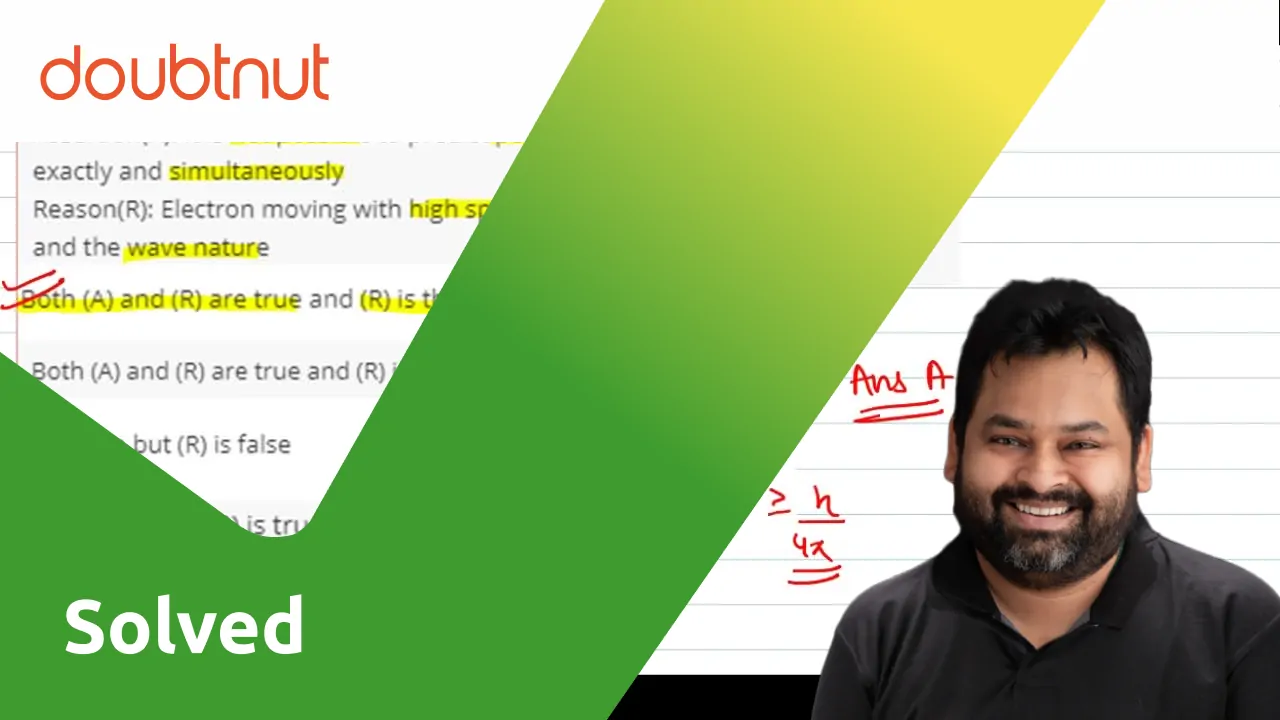Doubtnut App - Get 50 Lakh+ video solutions better than GoogleInstall Now

# Assertion(A): It is not possible to predict position and the velocity of an electron exactly and simultaneously Reason(R): Electron moving with high speed possesses both the particle nature and the wave nature

A

Both (A) and (R) are true and (R) is the correct explanation of (A)

B

Both (A) and (R) are true and (R) is not the correct explanation of (A)

C

(A) is true but (R) is false

D

(A) is false but (R) is true

Text Solution
Verified by Experts

Video Solution